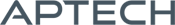# Discrete Choice Analysis Tools

Discrete Choice Analysis Tools

Availability: Out of stock

Discrete Choice Analysis Tools 2.0 provides an adaptable, efficient, and user-friendly environment for linear data classification.
Overview

Discrete Choice Analysis Tools 2.0 provides an adaptable, efficient, and user-friendly environment for linear data classification. It's designed with a full suite of tools built to accommodate individual model specificity, including adjustable parameter bounds, linear or nonlinear constraints, default or user-specified starting values, and user specified Gradient and Hessian procedures. Newly incorporated data and parameter input procedures make model set-up and implementation intuitive.

Platforms: Windows, Mac, and Linux

Requirements: GAUSS/GAUSS Engine/GAUSS Light v14 or higher

Features

## Binary and count models

• Binary probit
• Binary logit
• Negative binomial regression
• Poisson regression

## Multinomial logit models

• Conditional logit
• Nested logit
• Ordered logit
• Stereotype logit

## Logistic regression modelling

• L2/L1 regularized classifiers
• L2/L1-loss linear SVM

## Accessible, storable, and exportable output

• Parameter estimates
• Variance-covariance matrix for coefficient estimates and marginal effects
• Categorical dependent variables percentages
• Data descriptions of all independent variables
• Marginal effects of independent variables
• Predicted counts and residuals

## Model Selection and assessment

• Full model and restricted model log-likelihoods
• Chi-square statistic
• Agresti’s G-squared statistic
• Akaike information criterion (AIC)
• Bayesian information criterion (BIC)
• Likelihood ratio statistics and accompanying probability values
• McKelvey and Zovcina’s psuedo R-Squared
• Cragg and Uhler’s normed likelihood ratios
• Count R-Squared

Examples

Product Inquiry

6 + 7 = enter the result ex:(3 + 2 = 5)

﻿